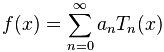# RooChebychev appears to draw the wrong shape

For part of my fit, I use a RooChebychev with one parameter. At the end of the fit, it comes out to be -0.950, which should describe a flat line at -0.950. However, when the fit is drawn it is above 0 and it definitely not constant. What is going on? Code snippets and plot follow.

``` RooRealVar b0("b0","Background: Chebychev b0",-0.950,-10000,10000); RooArgList bList(b0); RooChebychev bkg("bkg","Background", *mass, bList); ```[code] RooArgList shapes;
RooArgList yields;

RooAbsReal * nll = totalPdf.createNLL(*data,Extended(kTRUE));
RooMinuit m(*nll);
m.setVerbose(kFALSE);
RooFitResult *logfile = m.save(“logfile”,“Log File”);
m.minos();
m.minos();
[/code]
fit6.pdf (73.5 KB)

Hi,

Since one coefficient it is fixed by the normalisation, RooCgebychev with one parameter is a polynomial of degree 1 and not 0, i.e. it is not a constant.

Lorenzo

Thank you. Can you tell me how to extract this parameter?

Hi,

You pass in the constructor the parameter for RooChebychev (“b0” in your code example). There is no other parameter, since as I said, the offset is fixed by the normalisation.

Lorenzo

How can there be no other parameter? It’s still fitting to a polynomial, yes? Which should be described by an equation? I want the equation of the line it’s drawing.

You should be able to get the equation from the code implementing it
root.cern.ch/doc/v606/RooChebyc … tml#l00117

Lorenzo

Yes, it looks like it does not take a coefficient for the 0th cheby, that is, for 1, but just lets this be determined by the normalization, essentially making the 0th coefficient equal to the normalization factor. 2 questions:

1. Is there a way for me to call this normalization factor directly?
2. How does the normalization relate to the other coefficients? Put another way, is the equation norm1+b1T1+b2T2… or is it norm(1+b1T1+b2T2…) where bi are the coefficients and Ti are the polynomials?

Hi,

There is no way to get this normalisation factor. It is included in the variable definition of the polynomial, i.e x is redefined to be in the range [-1,1] and the polynomial are defined normalised in that range, as you can see in the code.

Lorenzo

I see that the range is mapped onto a different variable from -1 to 1, and I see the note that that is the form in which it is normalized, but you seem to be saying that the redefinition of the variable (that is, mapping it from -1 to 1) is the normalization, which cannot be true, at least not for the way the code redefines the variable. There has to be a normalization factor somewhere.

In any case, is there a place I can request this be changed? This seems needlessly obtuse. It seems better to just be able to add parameters according to the order polynomial you want, as here:bump

I suggest you to contact the RooFit author directly for this.